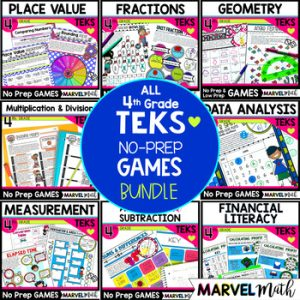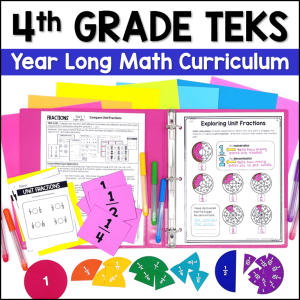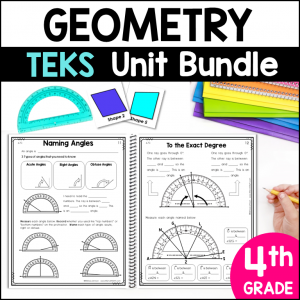Save 10% on your first order with code EXTRA10

\$5.00\$24.99187.25\$25.00
SKU: 3234830 Category:

## Description

Transform your math stations with this set of 11 no prep and low prep Geometry Games for 4th Grade that cover types of triangles, classifying quadrilaterals, protractor activities, geometry vocabulary, lines of symmetry and missing angles.

Your students will absolutely LOVE playing these games, and with repetitious play, they will internalize crucial geometry vocabulary. Games are a great way to practice skills and develop concepts AND they are amazing small group teaching tools.

Six of the games are completely no-prep, while the other 5 games have cards that will need to be cut apart.

Included Geometry Games:

• Geometry Guess Who: Analyzing attributes – parallel & perpendicular lines, acute, right and obtuse angles, naming triangles, types of quadrilaterals
• Aloha Attribute Race: parallel & perpendicular lines, acute, right and obtuse angles
• Attribute Battle Level 1: Classifying shapes, considering shape attributes
• Attribute Battle Level 2: Creating shapes with various attributes such as parallel & perpendicular lines, and specific types of angles
• Lines of Symmetry 4-in-a-Row: Determining the number of lines of symmetry in shapes
• Triangle Champion: Naming triangles based on attributes
• Quadrilateral Sort: Classifying quadrilaterals based on presence or absence of attributes
• Rolling Angles: Reading angles on a printed protractor
• Angle Fishing: Measuring angles using an actual protractor
• Spinning Adjacent Angles: Determine the measure of an unknown angle formed by two non-overlapping angles, given one or both angle measures

All Geometry Games come in 2 versions: Full Color and Blackline!

Students will stay far more engaged with games than with extra worksheets. Answer keys are included for the games that do not have random shape or number generation.

Standards Alignment:

TEKS:

4.6A – Identify points, lines, line segments, rays, angles, and perpendicular and parallel lines

4.6B – identify and draw one or more lines of symmetry, if they exist, for a two‐dimensional figure

4.6C – apply knowledge of right angles to identify acute, right, and obtuse triangles

4.6D – classify two‐dimensional figures based on the presence or absence of parallel or perpendicular lines or the presence or absence of angles of a specified size

4.7C – determine the approximate measures of angles in degrees to the nearest whole number using a protractor

4.7D – draw an angle with a given measure

4.7E – determine the measure of an unknown angle formed by two non‐overlapping adjacent angles given one or both angle measures

CCSS:

4.G.A.1 – Draw points, lines, line segments, rays, angles (right, acute, obtuse), and perpendicular and parallel lines. Identify these in two-dimensional figures.

4.G.A.2 – Classify two-dimensional figures based on the presence or absence of parallel or perpendicular lines, or the presence or absence of angles of a specified size. Recognize right triangles as a category, and identify right triangles.

4.G.A.3 – Recognize a line of symmetry for a two-dimensional figure as a line across the figure such that the figure can be folded along the line into matching parts. Identify line-symmetric figures and draw lines of symmetry.

4.MD.C.5 – Recognize angles as geometric shapes that are formed wherever two rays share a common endpoint, and understand concepts of angle measurement.

4.MD.C.6 – Measure angles in whole-number degrees using a protractor. Sketch angles of specified measure.

4.MD.C.7 – Recognize angle measure as additive. When an angle is decomposed into non-overlapping parts, the angle measure of the whole is the sum of the angle measures of the parts. Solve addition and subtraction problems to find unknown angles on a diagram in real world and mathematical problems, e.g., by using an equation with a symbol for the unknown angle measure.

This resource is a part of a time saving, money saving bundle:

4th Grade Geometry TEKS Unit and Bundle